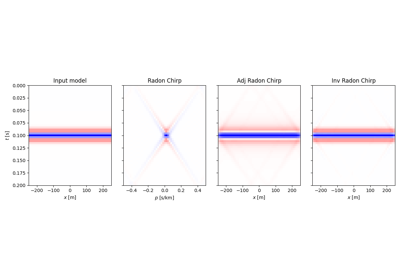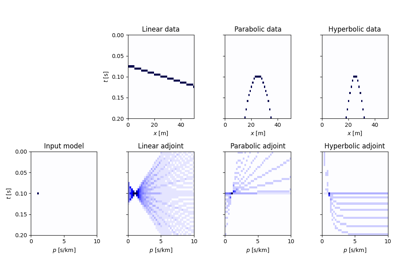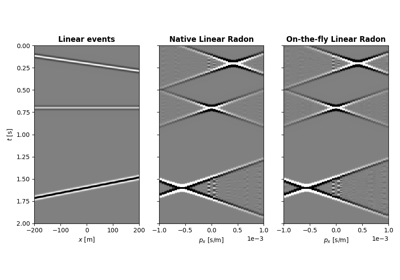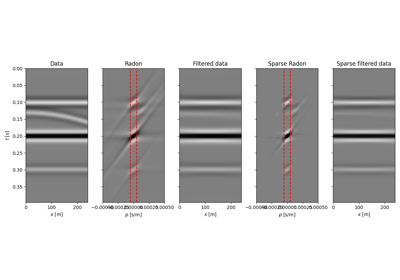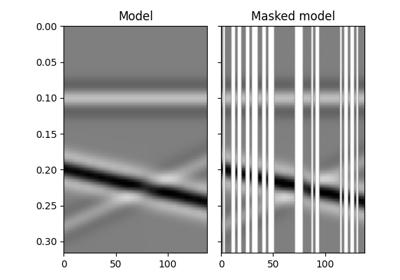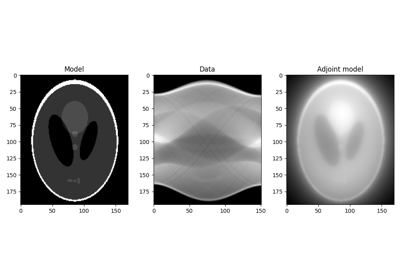pylops.signalprocessing.Radon2D(taxis, haxis, pxaxis, kind='linear', centeredh=True, interp=True, onthefly=False, engine='numpy', dtype='float64', name='R')[source]#

Apply two dimensional Radon forward (and adjoint) transform to a 2-dimensional array of size $$[n_{p_x} \times n_t]$$ (and $$[n_x \times n_t]$$).

In forward mode this entails to spreading the model vector along parametric curves (lines, parabolas, or hyperbolas depending on the choice of kind), while stacking values in the data vector along the same parametric curves is performed in adjoint mode.

Parameters
taxisnp.ndarray

Time axis

haxisnp.ndarray

Spatial axis

pxaxisnp.ndarray

Axis of scanning variable $$p_x$$ of parametric curve

kindstr, optional

Curve to be used for stacking/spreading (linear, parabolic, and hyperbolic are currently supported) or a function that takes $$(x, t_0, p_x)$$ as input and returns $$t$$ as output

centeredhbool, optional

Assume centered spatial axis (True) or not (False). If True the original haxis is ignored and a new axis is created.

interpbool, optional

Apply linear interpolation (True) or nearest interpolation (False) during stacking/spreading along parametric curve

ontheflybool, optional

Compute stacking parametric curves on-the-fly as part of forward and adjoint modelling (True) or at initialization and store them in look-up table (False). Using a look-up table is computationally more efficient but increases the memory burden

enginestr, optional

Engine used for computation (numpy or numba)

dtypestr, optional

Type of elements in input array.

namestr, optional

New in version 2.0.0.

Name of operator (to be used by pylops.utils.describe.describe)

Returns
r2oppylops.LinearOperator

Raises
KeyError

If engine is neither numpy nor numba

NotImplementedError

If kind is not linear, parabolic, or hyperbolic

pylops.signalprocessing.Radon3D

pylops.Spread

Notes

The Radon2D operator applies the following linear transform in adjoint mode to the data after reshaping it into a 2-dimensional array of size $$[n_x \times n_t]$$ in adjoint mode:

$m(p_x, t_0) = \int{d(x, t = f(p_x, x, t))} \,\mathrm{d}x$

where $$f(p_x, x, t) = t_0 + p_x x$$ where $$p_x = \sin(\theta)/v$$ in linear mode, $$f(p_x, x, t) = t_0 + p_x x^2$$ in parabolic mode, and $$f(p_x, x, t) = \sqrt{t_0^2 + x^2 / p_x^2}$$ in hyperbolic mode. Note that internally the $$p_x$$ axis will be normalized by the ratio of the spatial and time axes and used alongside unitless axes. Whilst this makes the linear mode fully unitless, users are required to apply additional scalings to the $$p_x$$ axis for other relationships:

• $$p_x$$ should be pre-multipled by $$d_x$$ for the parabolic relationship;

• $$p_x$$ should be pre-multipled by $$(d_t/d_x)^2$$ for the hyperbolic relationship.

As the adjoint operator can be interpreted as a repeated summation of sets of elements of the model vector along chosen parametric curves, the forward is implemented as spreading of values in the data vector along the same parametric curves. This operator is actually a thin wrapper around the pylops.Spread operator.

## Examples using pylops.signalprocessing.Radon2D#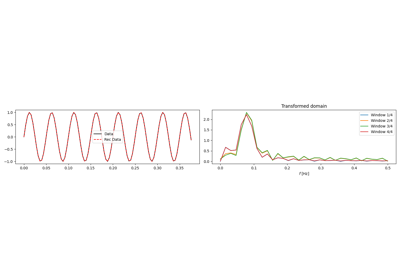1D, 2D and 3D Sliding

1D, 2D and 3D Sliding